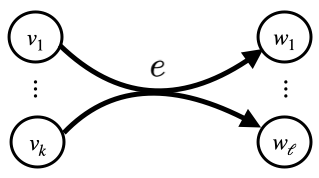## Overview

Signaling pathways are cornerstones of molecular and cellular biology. Hypergraphs and hyperpaths provide a precise model for signaling pathways but have been underutilized, due to their theoretical intractability and the lack of practical algorithms. The best previously-available method to find a shortest hyperpath, which is NP-complete, can only find acyclic hyperpaths, even though cycles are abundant in real signaling pathways.

## Methods

We give here source code for a new heuristic of finding shortest hyperpaths which for the first time may contain cycles. We also provide source code for our hyperpath enumeration algorithm, which exhaustively explores all possible s,t-hyperpaths.

Hyperpath heuristic
Our fast heuristic often finds a provably optimal hyperpath on real signaling pathways. Based on Dijkstra's algorithm for shortest paths in ordinary graphs, our hyperpath heuristic finds a possibly-cyclic hyperpath in under a minute, even on large cell-signaling hypergraphs with nearly 10,000 hyperedges.
Pathway enumeration algorithm
Our hyperpath enumeration algorithm maintains a queue of hyperpath problem instances consisting of insets and outsets. An outset for a problem instance is a set of hyperedges that are not allowed to be in the solution. An inset is a set of hyperedges that cannot be placed in the outset of child problem instances spawned from the current instance. Together, these sets ensure that the solution to a problem instance is ever computed twice.
Performance
On datasets from two common pathway databases, Reactome and NCI-PID, our hyperpath heuristic (in less than a minute of computation) finds a demonstrably optimal solution on 99.5% of all possible source, target instances. Furthermore, these experiments reveal the best prior methods, which were limited to acyclic hyperpaths, failed to find any solution for many instances where all source-target hyperpaths are in fact cyclic. For all such cyclic biological instances, our heuristic hyperpath is optimal.

## Source code

The source code is available on GitHub.